# Runge-Kutta

The Runge-Kutta methods are a set of numerical methods for solving first order differential equations of the form,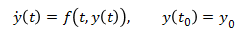They take the variable (y in the equation above) at a given time and use the known expression for the derivative of the variable to approximate the value of the variable a small interval of time, h, later in time. The simplest Runge-Kutta method is also known as the Euler method which is what the Example Problem used. It uses the following expression to approximate what y will be after the time interval h.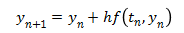The Euler method is simple since it is only the first order Runge-Kutta, but it has poor accuracy. Much better accuracy can be obtained using the same step size, h, by using the following fourth order Runge-Kutta method. This fourth order Runge-Kutta method is probably the most commonly used Runge-Kutta method, as it it a good balance between accuracy and complexity.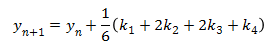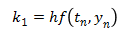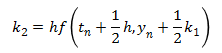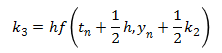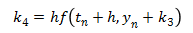This next method is a version of the fifth order Runge-Kutta method. (There are several different versions of the higher order methods.) It is more accurate than the fourth order Runge-Kutta method, although it is also more complex.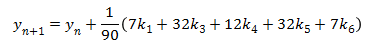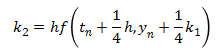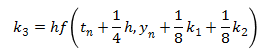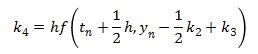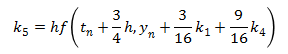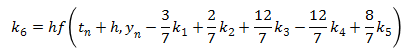The trebuchet simulation uses the fifth order Runge-Kutta method to solve the Equations of Motion. It also uses the fourth order Runge-Kutta method to check the accuracy of the solution. Any numerical method only gives an approximation of the actual answer, and its accuracy is dependent on the step size, h. The way the simulation determines it is using a small enough step size is that it compares the answers of the fourth and fifth order methods to make sure the answers agree within a specified tolerance of each other. If the answers are very close then the answer is considered to be a reasonable approximation, but if they aren’t close, the time step is made smaller until the answers do agree within the tolerance.

 Units English (feet)English (inches)Metric Length of Short Arm ft Length of Long Arm ft Length of Sling ft Length of Weight ft Height of Pivot ft Uniform arm Mass of Arm lb Inertia of Arm lb·ft² Pivot to Arm CG ft Mass of Weight lb Inertia of Weight lb·ft² Projectile BaseballGolf BallPumpkinCowCustom Mass of Projectile lb Projectile Diameter ft Wind Speed ft/s Release Angle ° Play speed: 1x Link to save this trebuchet

## VirtualTrebuchet 2.0

VirtualTrebuchet is a web based trebuchet simulator that will allow you to quickly evaluate different trebuchet configurations.

1. To begin, enter the parameters of your trebuchet in the input boxes.
2. Next, press the "Simulate" button located under the inputs.
3. Finally, watch your trebuchet go.